Function Repository Resource:

# NineJSymbol

Evaluate the Wigner 9-j symbol

Contributed by: Jan Mangaldan
 ResourceFunction["NineJSymbol"][{{j1,j2,j3},{j4,j5,j6},{j7,j8,j9}}] gives the values of the Wigner 9‐j symbol.

## Details

The 9‐j symbols vanish except when certain triples of the ji satisfy triangle inequalities.
The parameters of ResourceFunction["NineJSymbol"] can be integers or half‐integers.

## Examples

### Basic Examples (1)

Evaluate numerically:

 In:=Out=### Scope (3)

NineJSymbol works with integer and half‐integer arguments:

 In:=Out=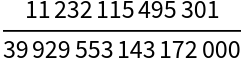Evaluate for large arguments:

 In:=Out=Evaluate for inexact arguments:

 In:=Out=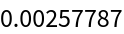### Properties and Relations (3)

NineJSymbol is invariant under transposition:

 In:=Out=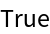NineJSymbol is invariant under an even permutation of its rows or columns:

 In:=Out=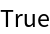In:=Out=Under an odd permutation of its rows or columns, NineJSymbol gains an extra phase factor:

 In:=Out=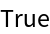In:=Out=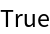When one of the entries is 0, NineJSymbol can be expressed in terms of SixJSymbol:

 In:=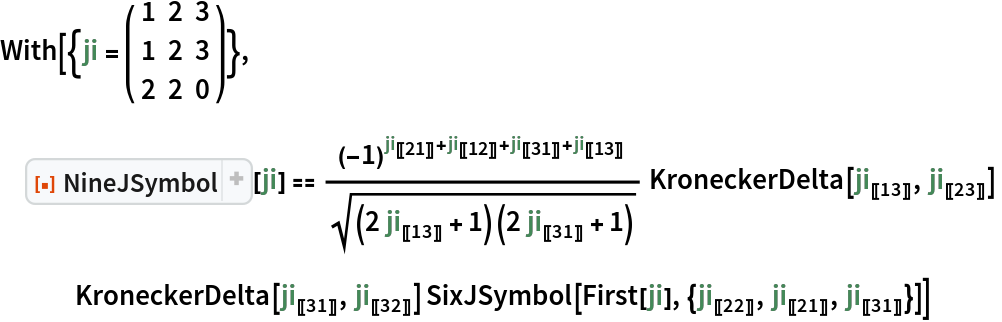Out=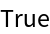### Possible Issues (1)

A message is issued and the result 0 returned for unphysical cases:

 In:=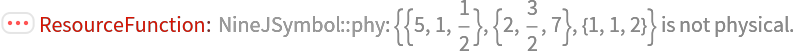Out=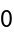## Version History

• 1.0.2 – 20 September 2023
• 1.0.1 – 03 March 2021
• 1.0.0 – 26 January 2021